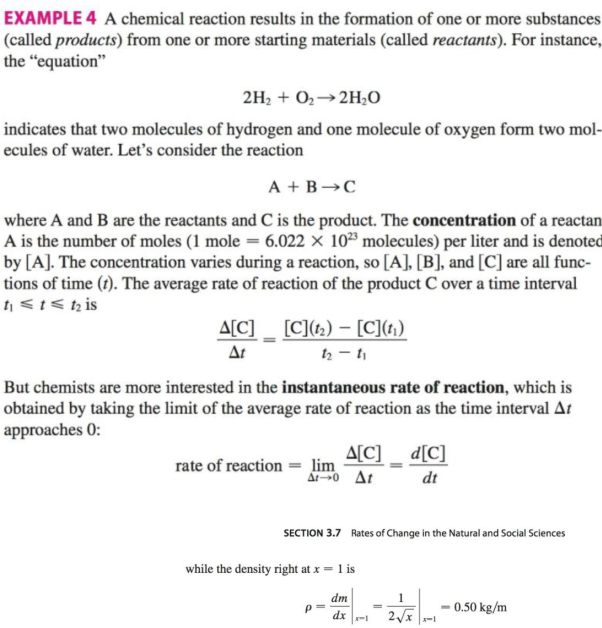Chapter 3.7, Problem 24E### Single Variable Calculus: Early Tr...

8th Edition
James Stewart
ISBN: 9781305270343

#### Solutions

Chapter
Section### Single Variable Calculus: Early Tr...

8th Edition
James Stewart
ISBN: 9781305270343
Textbook Problem

# If, in Example 4, one molecule of the product C is formed from one molecule of the reactant A and one molecule of the reactant B, and the initial concentrations of A and B have a common value [A] = [B] = a moles/ L, then[C] = a2kt/(akt + 1)where k is a constant.(a) Find the rate of reaction at time t.(b) Show that if x = [C], then d x d t = k ( a − x ) 2 c) What happens to the concentration as t → ∞?(d) What happens to the rate of reaction as t →4 ∞?(e) What do the results of parts (c) and (d) mean in practical terms?EXAMPLE 4(a)

To determine

To find: The rate of reaction at time t.

Explanation

Given:

The expression is given as below.

[C]=a2ktakt+1 (1)

Here, the variable [C] is the product, and a is the initial concentration of [A]=[B] in moles/L.

Calculation:

Differentiate equation (1) with respect t.

d[C]dt=[(akt+1)(a2k)](a2kt)(ak)(akt+

(b)

To determine

To show: The equation dxdt=k(ax)2, where [C]=x.

(c)

To determine

To show: The concentration if time t tends to .

(d)

To determine

To find: The rate of reaction if time t tends to .

(e)

To determine

To compare: The practical means of part (c) and (d).

### Still sussing out bartleby?

Check out a sample textbook solution.

See a sample solution

#### The Solution to Your Study Problems

Bartleby provides explanations to thousands of textbook problems written by our experts, many with advanced degrees!

Get Started

#### In Exercises 31-34, evaluate h(2), where h = g f. 32. f(x)=x213;g(x)=3x3+1

Applied Calculus for the Managerial, Life, and Social Sciences: A Brief Approach

#### Calculate y'. 5. y = x2 sin x

Single Variable Calculus: Early Transcendentals

#### 0 1 π It does not exist.

Study Guide for Stewart's Multivariable Calculus, 8th